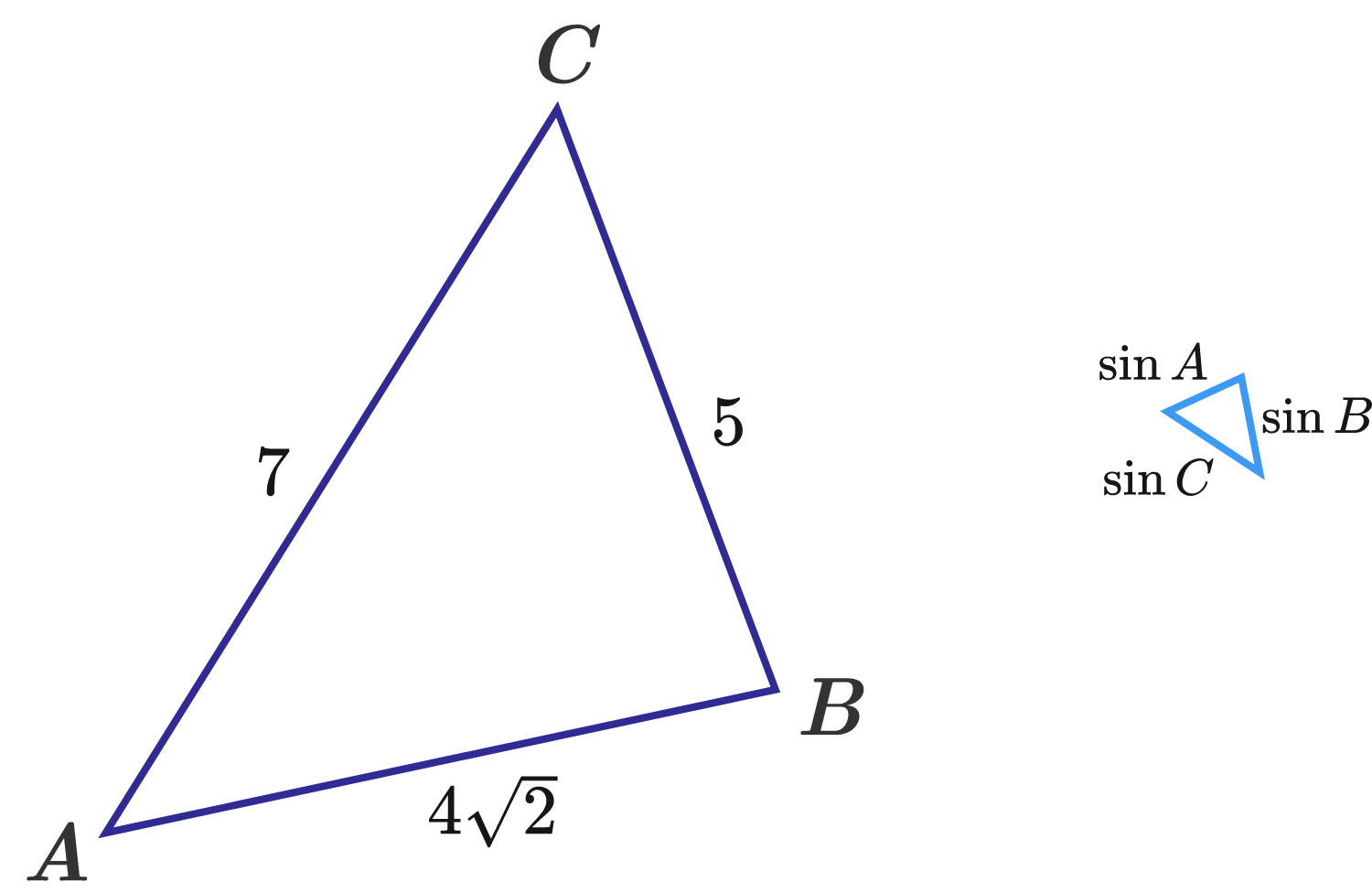Geometry Level 3

$ABC$ is a triangle with side lengths $5,$ $4\sqrt2,$ and $7.$

Another triangle has side lengths $\sin A,$ $\sin B,$ and $\sin C.$

If the area of that triangle is $\frac{p}{q},$ where $p$ and $q$ are coprime positive integers, then what is $p+q?$×